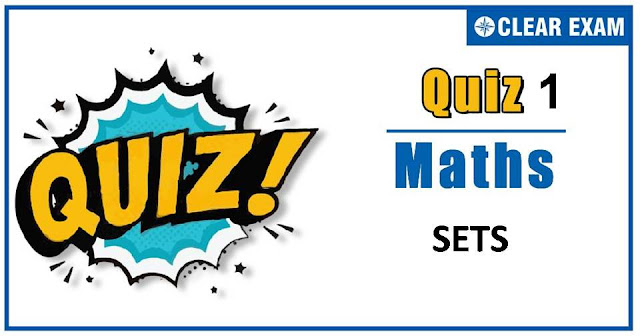## [LATEST]\$type=sticky\$show=home\$rm=0\$va=0\$count=4\$va=0

As per analysis for previous years, it has been observed that students preparing for JEE MAINS find Mathematics out of all the sections to be complex to handle and the majority of them are not able to comprehend the reason behind it. This problem arises especially because these aspirants appearing for the examination are more inclined to have a keen interest in Mathematics due to their ENGINEERING background.

Furthermore, sections such as Mathematics are dominantly based on theories, laws, numerical in comparison to a section of Engineering which is more of fact-based, Physics, and includes substantial explanations. By using the table given below, you easily and directly access to the topics and respective links of MCQs. Moreover, to make learning smooth and efficient, all the questions come with their supportive solutions to make utilization of time even more productive. Students will be covered for all their studies as the topics are available from basics to even the most advanced.

Q2.In a class of 35 students, 17 have taken Mathematics, 10 have taken Mathematics but not Economics.
If each student has taken either Mathematics or Economics or both, then
the number of students who have taken Economics but not Mathematics is
•  18
•  25
•  7
•  32

Q3.  Let R1 be a relation defined by R1={(a,b)│a≥b,a,b∈R}. Then, R1 is
•  Reflexive, transitive but not symmetric
•  An equivalence relation on R
•  Symmetric, transitive but not reflexive
•  Neither transitive not reflexive but symmetric
Solution
For any a∈R, we have a≥a Therefore, the relation R is reflexive. R is not symmetric as (2,1)∈R but (1,2)∉R. The relation R is transitive also, because (a,b)∈R,(b,c)∈R imply that a≥b and b≥c which in turn imply that a≥c

Q4. If two sets A and B are having 99 elements in common, then the number of elements common to each of the sets A×B and B×A are
•  299
•  992
•  100
•  18
Solution
∵ Number of elements common to each set is 99×99=992
Q5.If X={4n-3n-1∶n∈N} and Y={9(n-1):n∈N}, then X∪Y is equal to
•  X
•  None of these
•  N
•  Y
Solution
Q6. If A and B are two sets such that n(A)=7,n(B)=6 and (A∩B)≠ϕ. Then the greatest possible value of         n(A Δ B), is
•  12
•  11
•  13
•  10
Solution

Q7.An investigator interviewed 100 students to determine the performance of three drinks milk,
coffee and tea. The investigator reported that 10 students take all three drinks milk, coffee
and tea; 20 students take milk and coffee, 30 students take coffee and tea, 25 students take
mile and tea, 12 students take milk only, 5 students take coffee only and 8 students take tea only.
Then, the number of students who did not take any of the three drinks, is
• 10
•  25
•  20
•  30
Solution

Q8.If R={(a,b):|a+b|=a+b} is a relation defined on a set{-1,0,1}, then R is
•   Reflexive
•   Anti symmetric
•   Symmetric
•  Transitive
Solution

Q9.If sets A and B are defined as A={(x,y):y=1/x,0≠x∈R}, B={(x,y):y=-x,x∈R}, then
•  A∩B=ϕ
•  A∩B=B
•   A∩B=A
•  None of these
Solution
The set A is the set of all points on the hyperbola xy=1 having its two branches in the first
and third quadrants, while the set B is the set of all points on y=-x which
lies in second and four quadrants. These two curves do not intersect. Hence, A∩B=ϕ.

Q10. If R is a relation from a set A to a set B and S is a relation from B to a setC, then the relation SoR
•  Is from A to C
•  Is from C to A
•  Does not exist
•  None of these
Solution
No solution available#### Written by: AUTHORNAME

AUTHORDESCRIPTION## Want to know more

Please fill in the details below:

## Latest NEET Articles\$type=three\$c=3\$author=hide\$comment=hide\$rm=hide\$date=hide\$snippet=hide

Name

ltr
item
BEST NEET COACHING CENTER | BEST IIT JEE COACHING INSTITUTE | BEST NEET, IIT JEE COACHING INSTITUTE: SETS-QUIZ-1
SETS-QUIZ-1
https://1.bp.blogspot.com/-KlnFNMpVCgY/X5JR_2dLPnI/AAAAAAAAE_w/n7xNVQnD_R4Xns-WmjE8udceEqALmpAVACLcBGAsYHQ/w640-h336/quiz.jpg
https://1.bp.blogspot.com/-KlnFNMpVCgY/X5JR_2dLPnI/AAAAAAAAE_w/n7xNVQnD_R4Xns-WmjE8udceEqALmpAVACLcBGAsYHQ/s72-w640-c-h336/quiz.jpg
BEST NEET COACHING CENTER | BEST IIT JEE COACHING INSTITUTE | BEST NEET, IIT JEE COACHING INSTITUTE
https://www.cleariitmedical.com/2020/10/SETS-QUIZ-1.html
https://www.cleariitmedical.com/
https://www.cleariitmedical.com/
https://www.cleariitmedical.com/2020/10/SETS-QUIZ-1.html
true
7783647550433378923
UTF-8